# Logistic 回归模型

Logistic 回归是一种广义的回归模型，其与多元线性回归有着很多相似之处，模型的形式基本相同，虽然也被称为回归，但是其更多的情况使用在分类问题上，同时又以二分类更为常用。

## 模型形式

Logistic 回归的模型形式和线性回归一样，都是 y = wx + b，其中 x 可以是一个多维的特征，唯一不同的地方在于 Logistic 回归会对 y 作用一个 logistic 函数，将其变为一种概率的结果。 Logistic 函数作为 Logistic 回归的核心，我们下面讲一讲 Logistic 函数，也被称为 Sigmoid 函数。

### Sigmoid 函数

Sigmoid 函数非常简单，其公式如下

$$f(x) = \frac{1}{1 + e^{-x}}$$

Sigmoid 函数的图像如下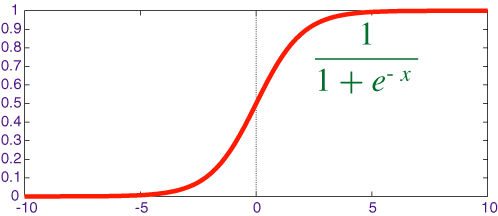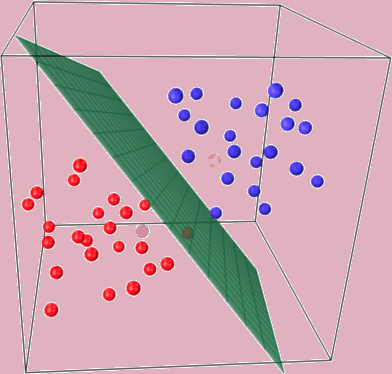## 回归问题 vs 分类问题

Logistic 回归处理的是一个分类问题，而上一个模型是回归模型，那么回归问题和分类问题的区别在哪里呢？

## 损失函数

Logistic 回归使用了 Sigmoid 函数将结果变到 0 ~ 1 之间，对于任意输入一个数据，经过 Sigmoid 之后的结果我们记为 $\hat{y}$，表示这个数据点属于第二类的概率，那么其属于第一类的概率就是 $1-\hat{y}$。如果这个数据点属于第二类，我们希望 $\hat{y}$ 越大越好，也就是越靠近 1 越好，如果这个数据属于第一类，那么我们希望 $1-\hat{y}$ 越大越好，也就是 $\hat{y}$ 越小越好，越靠近 0 越好，所以我们可以这样设计我们的 loss 函数

$$loss = -(y log(\hat{y}) + (1 - y) log(1 - \hat{y}))$$

$$loss = - (log(1 - \hat{y}))$$

$$loss = -(log(\hat{y}))$$

import torch
import numpy as np
import matplotlib.pyplot as plt
%matplotlib inline

# 设定随机种子
torch.manual_seed(2017)

<torch._C.Generator at 0x108f3c5f0>


# 从 data.txt 中读入点
with open('./data.txt', 'r') as f:
data_list = [i.split('\n').split(',') for i in f.readlines()]
data = [(float(i), float(i), float(i)) for i in data_list]

# 标准化
x0_max = max([i for i in data])
x1_max = max([i for i in data])
data = [(i/x0_max, i/x1_max, i) for i in data]

x0 = list(filter(lambda x: x[-1] == 0.0, data)) # 选择第一类的点
x1 = list(filter(lambda x: x[-1] == 1.0, data)) # 选择第二类的点

plot_x0 = [i for i in x0]
plot_y0 = [i for i in x0]
plot_x1 = [i for i in x1]
plot_y1 = [i for i in x1]

plt.plot(plot_x0, plot_y0, 'ro', label='x_0')
plt.plot(plot_x1, plot_y1, 'bo', label='x_1')
plt.legend(loc='best')

<matplotlib.legend.Legend at 0x108137c50>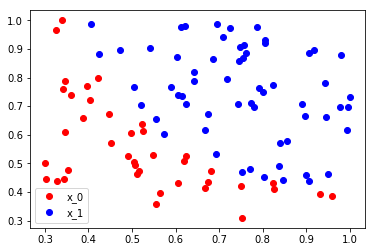np_data = np.array(data, dtype='float32') # 转换成 numpy array
x_data = torch.from_numpy(np_data[:, 0:2]) # 转换成 Tensor, 大小是 [100, 2]
y_data = torch.from_numpy(np_data[:, -1]).unsqueeze(1) # 转换成 Tensor，大小是 [100, 1]


$$f(x) = \frac{1}{1 + e^{-x}}$$

# 定义 sigmoid 函数
def sigmoid(x):
return 1 / (1 + np.exp(-x))


# 画出 sigmoid 的图像

plot_x = np.arange(-10, 10.01, 0.01)
plot_y = sigmoid(plot_x)

plt.plot(plot_x, plot_y, 'r')

[<matplotlib.lines.Line2D at 0x10be61908>]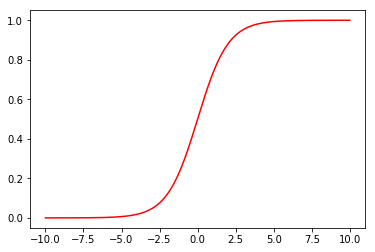x_data = Variable(x_data)
y_data = Variable(y_data)


import torch.nn.functional as F

# 定义 logistic 回归模型

def logistic_regression(x):
return F.sigmoid(torch.mm(x, w) + b)


# 画出参数更新之前的结果
w0 = w.data
w1 = w.data
b0 = b.data

plot_x = np.arange(0.2, 1, 0.01)
plot_y = (-w0 * plot_x - b0) / w1

plt.plot(plot_x, plot_y, 'g', label='cutting line')
plt.plot(plot_x0, plot_y0, 'ro', label='x_0')
plt.plot(plot_x1, plot_y1, 'bo', label='x_1')
plt.legend(loc='best')

<matplotlib.legend.Legend at 0x10bf66c18>$$loss = -(y log(\hat{y}) + (1 - y) log(1 - \hat{y}))$$

# 计算loss
def binary_loss(y_pred, y):
logits = (y * y_pred.clamp(1e-12).log() + (1 - y) * (1 - y_pred).clamp(1e-12).log()).mean()
return -logits


y_pred = logistic_regression(x_data)
loss = binary_loss(y_pred, y_data)
print(loss)

Variable containing:
0.6412
[torch.FloatTensor of size 1]


# 自动求导并更新参数
loss.backward()
w.data = w.data - 0.1 * w.grad.data
b.data = b.data - 0.1 * b.grad.data

# 算出一次更新之后的loss
y_pred = logistic_regression(x_data)
loss = binary_loss(y_pred, y_data)
print(loss)

Variable containing:
0.6407
[torch.FloatTensor of size 1]


# 使用 torch.optim 更新参数
from torch import nn
w = nn.Parameter(torch.randn(2, 1))
b = nn.Parameter(torch.zeros(1))

def logistic_regression(x):
return F.sigmoid(torch.mm(x, w) + b)

optimizer = torch.optim.SGD([w, b], lr=1.)

# 进行 1000 次更新
import time

start = time.time()
for e in range(1000):
# 前向传播
y_pred = logistic_regression(x_data)
loss = binary_loss(y_pred, y_data) # 计算 loss
# 反向传播
loss.backward()
optimizer.step() # 使用优化器来更新参数
# 计算正确率
acc = (mask == y_data).sum().data / y_data.shape
if (e + 1) % 200 == 0:
print('epoch: {}, Loss: {:.5f}, Acc: {:.5f}'.format(e+1, loss.data, acc))
during = time.time() - start
print()
print('During Time: {:.3f} s'.format(during))

epoch: 200, Loss: 0.39730, Acc: 0.92000
epoch: 400, Loss: 0.32458, Acc: 0.92000
epoch: 600, Loss: 0.29065, Acc: 0.91000
epoch: 800, Loss: 0.27077, Acc: 0.91000
epoch: 1000, Loss: 0.25765, Acc: 0.90000

During Time: 0.595 s


# 画出更新之后的结果
w0 = w.data
w1 = w.data
b0 = b.data

plot_x = np.arange(0.2, 1, 0.01)
plot_y = (-w0 * plot_x - b0) / w1

plt.plot(plot_x, plot_y, 'g', label='cutting line')
plt.plot(plot_x0, plot_y0, 'ro', label='x_0')
plt.plot(plot_x1, plot_y1, 'bo', label='x_1')
plt.legend(loc='best')

<matplotlib.legend.Legend at 0x10c08ec50>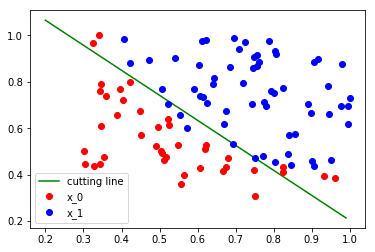PyTorch 为我们实现的 loss 函数有两个好处，第一是方便我们使用，不需要重复造轮子，第二就是其实现是在底层 C++ 语言上的，所以速度上和稳定性上都要比我们自己实现的要好

# 使用自带的loss
criterion = nn.BCEWithLogitsLoss() # 将 sigmoid 和 loss 写在一层，有更快的速度、更好的稳定性

w = nn.Parameter(torch.randn(2, 1))
b = nn.Parameter(torch.zeros(1))

def logistic_reg(x):

optimizer = torch.optim.SGD([w, b], 1.)

y_pred = logistic_reg(x_data)
loss = criterion(y_pred, y_data)
print(loss.data)

 0.6363
[torch.FloatTensor of size 1]

# 同样进行 1000 次更新

start = time.time()
for e in range(1000):
# 前向传播
y_pred = logistic_reg(x_data)
loss = criterion(y_pred, y_data)
# 反向传播
loss.backward()
optimizer.step()
# 计算正确率
acc = (mask == y_data).sum().data / y_data.shape
if (e + 1) % 200 == 0:
print('epoch: {}, Loss: {:.5f}, Acc: {:.5f}'.format(e+1, loss.data, acc))

during = time.time() - start
print()
print('During Time: {:.3f} s'.format(during))

epoch: 200, Loss: 0.39538, Acc: 0.88000
epoch: 400, Loss: 0.32407, Acc: 0.87000
epoch: 600, Loss: 0.29039, Acc: 0.87000
epoch: 800, Loss: 0.27061, Acc: 0.87000
epoch: 1000, Loss: 0.25753, Acc: 0.88000

During Time: 0.527 s